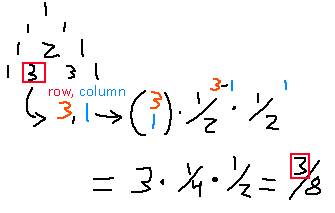# Pascal Triangle Creator

One way to generate Pascal's triangle is by using the combination function, and taking powers of halves and multiplying them together. Below is an example:Using the fields below, you can change the weights of the fractions to create a skewed version of the triangle!

The two numbers you put in are the numerators of a fraction. The sum of the two numbers you put in will be the denominators, so you'll always end up with a sum of 1 in the end. Getting the original triangle means putting in the same values in the fields.

Rows to generate:
Fraction numerator 1:
Fraction numerator 2:

Oh. I didn't set an upper limit on how many rows you can generate. Don't pick a number higher than 70, for the sake of your own computer.